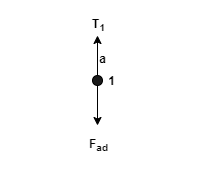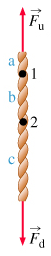# Problem: To understand the concept of tension and the relationship between tension and force. This problem introduces the concept of tension. The example is a rope, oriented vertically, that is being pulled from both ends. (Figure 1) Let Fu and Fd (with u for up and d for down) represent the magnitude of the forces acting on the top and bottom of the rope, respectively. Assume that the rope is massless so that its weight is negligible compared with the tension. (This is not a ridiculous approximation-modern rope materials such as Kevlar can carry tensions thousands of times greater than the weight of tens of meters of such rope.) Consider the three sections of rope labeled a, b, and c in the figure. At point 1, a downward force of magnitude Fad acts on section a. At point 1, an upward force of magnitude Fbu acts on section b. At point 1, the tension in the rope is T1. At point 2, a downward force of magnitude Fbd acts on section b. At point 2, an upward force of magnitude Fcu acts on section c. At point 2, the tension in the rope is T2. Assume, too, that the rope is at equilibrium.Part AWhat is the magnitude Fad of the downward force on section a?Express your answer in terms of the tension T1.Part BWhat is the magnitude Fbu of the upward force on section b?Express your answer in terms of the tension T1.Part CThe magnitude of the upward force on c, Fcu, and the magnitude of the downward force on b, Fbd, are equal because of which of Newton's laws?Part DThe magnitude of the force Fbu is ____ Fbd.Part ENow consider the forces on the ends of the rope. What is the relationship between the magnitudes of these two forces?Now consider the forces on the ends of the rope. What is the relationship between the magnitudes of these two forces?a) Fu &gt; Fdb) Fu &lt; Fdc) Fu = FdPart FThe ends of a massless rope are attached to two stationary objects (e.g., two trees or two cars) so that the rope makes a straight line. For this situation, which of the following statements are true?Check all that apply.a) The tension in the rope is everywhere the same.b) The magnitudes of the forces exerted on the two objects by the rope are the same.c) The forces exerted on the two objects by the rope must be in opposite directions.d) The forces exerted on the two objects by the rope must be in the direction of the rope

###### FREE Expert Solution

Take up as positive and down as negative.

Part AThe acceleration is zero at equilibrium.

ΣF = ma

88% (463 ratings)###### Problem Details

To understand the concept of tension and the relationship between tension and force. This problem introduces the concept of tension. The example is a rope, oriented vertically, that is being pulled from both ends. (Figure 1)

Let Fu and Fd (with u for up and d for down) represent the magnitude of the forces acting on the top and bottom of the rope, respectively. Assume that the rope is massless so that its weight is negligible compared with the tension. (This is not a ridiculous approximation-modern rope materials such as Kevlar can carry tensions thousands of times greater than the weight of tens of meters of such rope.)

Consider the three sections of rope labeled a, b, and c in the figure.

At point 1, a downward force of magnitude Fad acts on section a.

At point 1, an upward force of magnitude Fbu acts on section b.

At point 1, the tension in the rope is T1

At point 2, a downward force of magnitude Fbd acts on section b.

At point 2, an upward force of magnitude Fcu acts on section c.

At point 2, the tension in the rope is T2. Assume, too, that the rope is at equilibrium.Part A

What is the magnitude Fad of the downward force on section a?

Part B

What is the magnitude Fbu of the upward force on section b?

Part C

The magnitude of the upward force on c, Fcu, and the magnitude of the downward force on b, Fbd, are equal because of which of Newton's laws?

Part D

The magnitude of the force Fbu is ____ Fbd.

Part E

Now consider the forces on the ends of the rope. What is the relationship between the magnitudes of these two forces?

Now consider the forces on the ends of the rope. What is the relationship between the magnitudes of these two forces?

a) Fu > Fd

b) Fu < Fd

c) Fu = Fd

Part F

The ends of a massless rope are attached to two stationary objects (e.g., two trees or two cars) so that the rope makes a straight line. For this situation, which of the following statements are true?

Check all that apply.

a) The tension in the rope is everywhere the same.

b) The magnitudes of the forces exerted on the two objects by the rope are the same.

c) The forces exerted on the two objects by the rope must be in opposite directions.

d) The forces exerted on the two objects by the rope must be in the direction of the rope